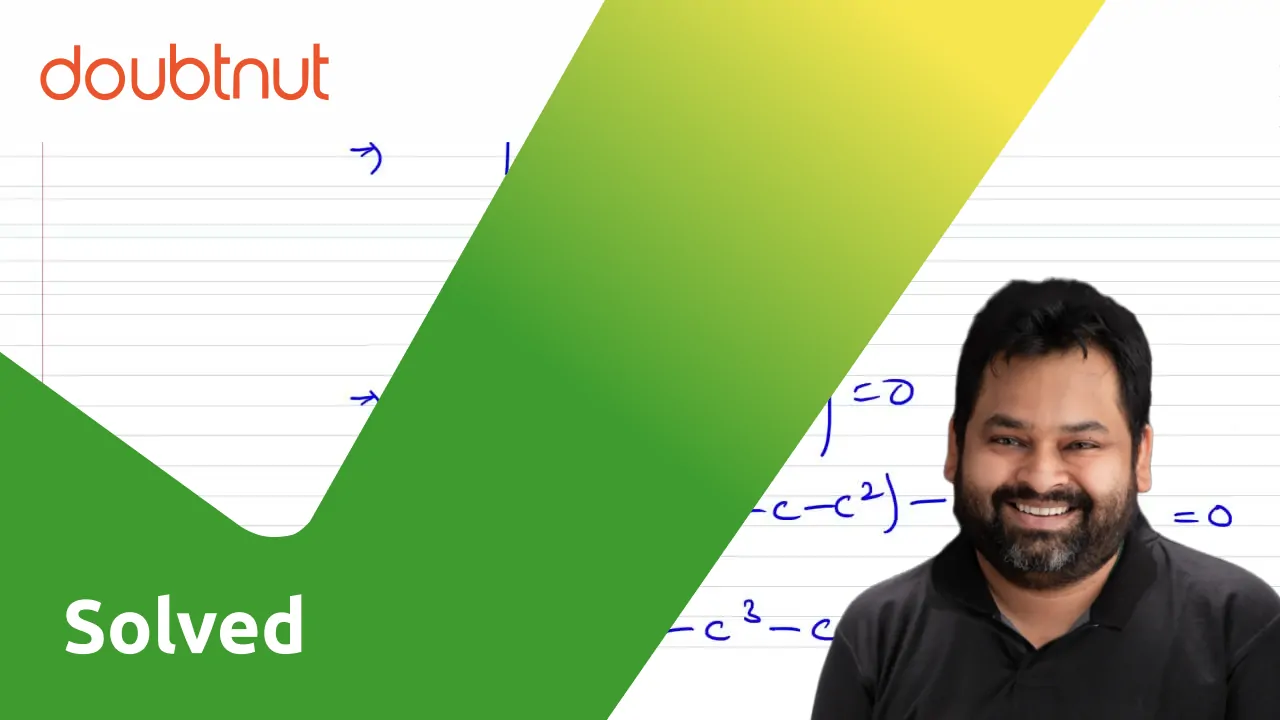Doubtnut App - Get 50 Lakh+ video solutions better than GoogleInstall Now

# The greatest value of c∈R for which the system of linear equations x-cy-cz = 0, cx -y + cz =0, cx + cy-z =0 has a non-trivial solution, is

A

1

B

12

C

2

D

0

Text Solution
Verified by Experts

## Key Idea A homogeneous system of linear equations have non-trivial solutions iff Δ=0 Given system of linear equations is x−cy−cz=0 cx−y+cz=0 and cx+cy−z=0 We know that a homogeneous system of linear equations have non-trivial solutions iff Δ=0 ⇒∣∣ ∣∣1−c−cc−1ccc−1∣∣ ∣∣=0 ⇒1(1−c2)+c(−c−c2)−c(c2+c)=0 ⇒1−c2−c2−c3−c3−c2=0 ⇒−2c3−3c2+1=0 ⇒2c3+3c2−1=0 ⇒(c+1)[2c2+c−1]=0 ⇒(c+1)[2c2+2c−c−1]=0 ⇒(c+1)(2c−1)(c+1)=0 ⇒c=−1or12 Clearly, the greatest value of c is 12.